# Multiway search trees 1 Outline In this topic

• Slides: 59
Download presentationMultiway search trees 1 Outline In this topic we will look at: – – – In-order traversals of binary search trees Limitations of in-order traversals with n-ary trees An introduction to multiway search trees An implementation in C++ In-order traversals of multiway trees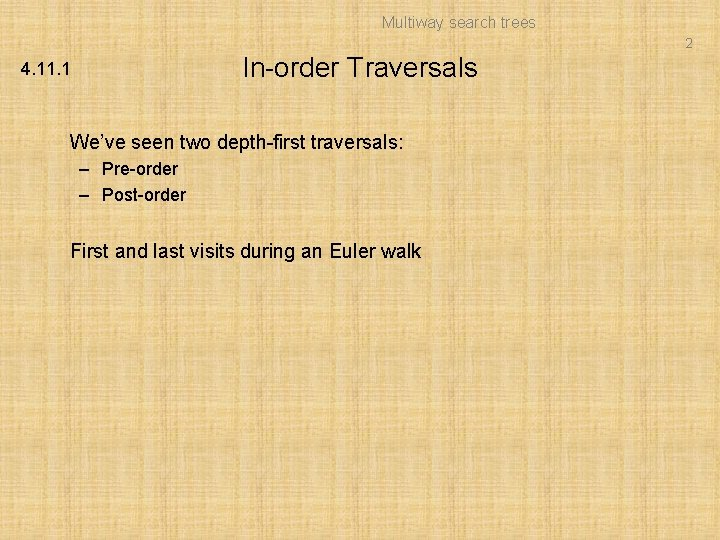Multiway search trees 2 In-order Traversals 4. 11. 1 We’ve seen two depth-first traversals: – Pre-order – Post-order First and last visits during an Euler walkMultiway search trees 3 4. 11. 1 In-order Traversals For binary trees, there is a third intermediate visit – An in-order depth-first traversal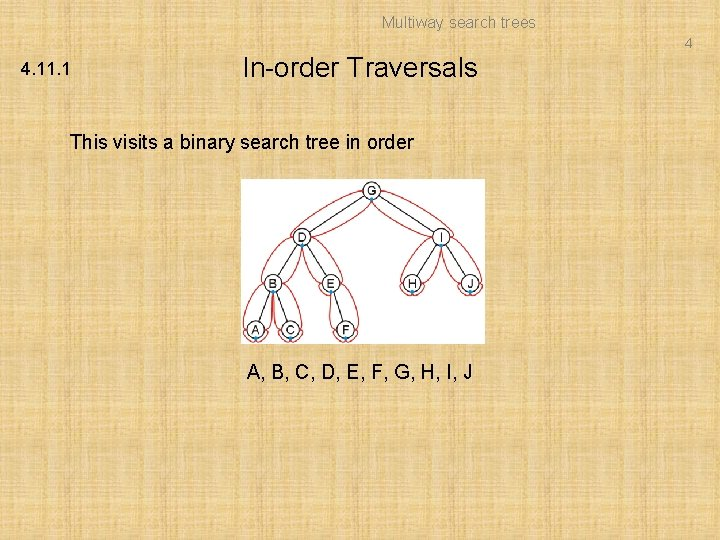Multiway search trees 4 4. 11. 1 In-order Traversals This visits a binary search tree in order A, B, C, D, E, F, G, H, I, J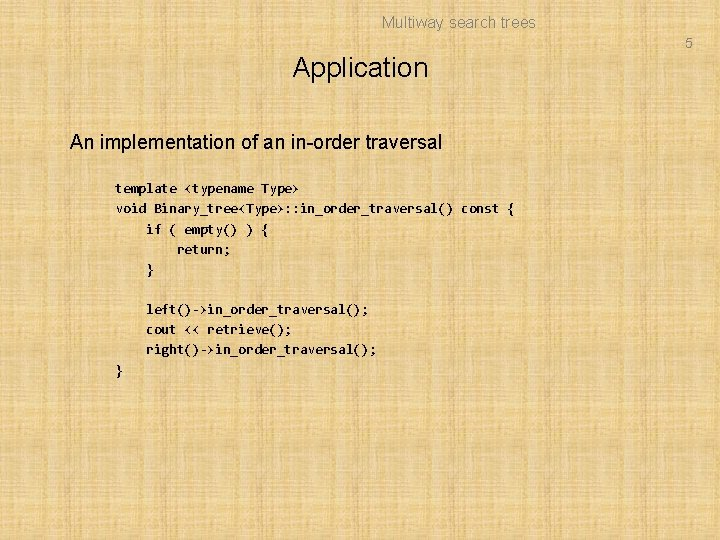Multiway search trees 5 Application An implementation of an in-order traversal template void Binary_tree: : in_order_traversal() const { if ( empty() ) { return; } left()->in_order_traversal(); cout << retrieve(); right()->in_order_traversal(); }Multiway search trees 6 4. 11. 1. 1 In-order traversals on expression trees Printing an expression tree (pretty printing or human-readable printing) using in-fix notation requires an in-order traversal (3 x + 5 + y)(z + 7)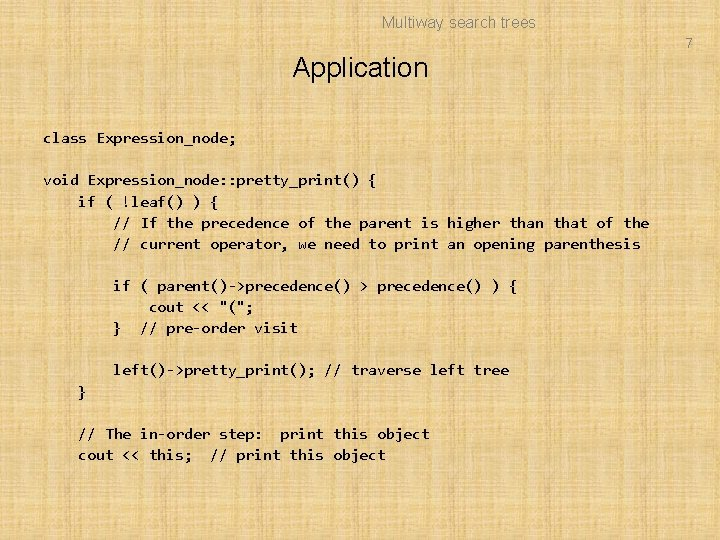Multiway search trees 7 Application class Expression_node; void Expression_node: : pretty_print() { if ( !leaf() ) { // If the precedence of the parent is higher than that of the // current operator, we need to print an opening parenthesis if ( parent()->precedence() > precedence() ) { cout << "("; } // pre-order visit left()->pretty_print(); // traverse left tree } // The in-order step: print this object cout << this; // print this object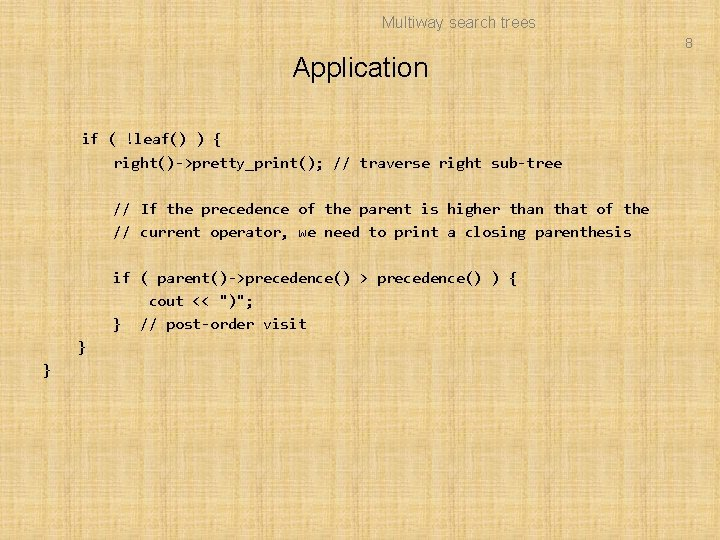Multiway search trees 8 Application if ( !leaf() ) { right()->pretty_print(); // traverse right sub-tree // If the precedence of the parent is higher than that of the // current operator, we need to print a closing parenthesis if ( parent()->precedence() > precedence() ) { cout << ")"; } // post-order visit } }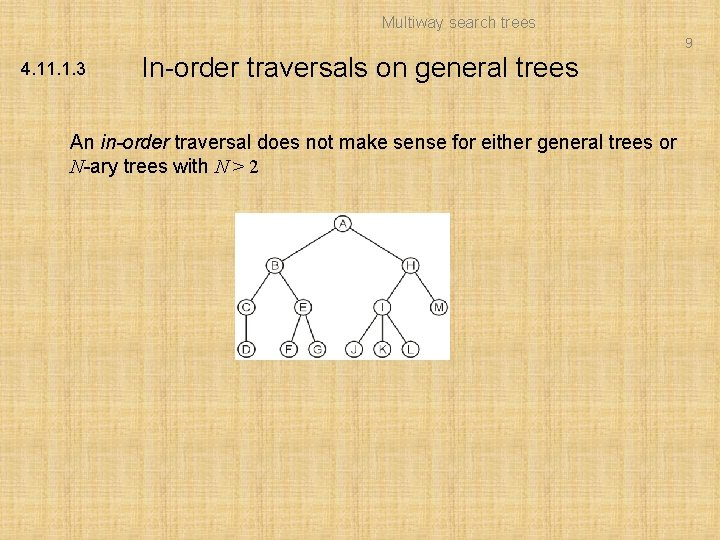Multiway search trees 9 4. 11. 1. 3 In-order traversals on general trees An in-order traversal does not make sense for either general trees or N-ary trees with N > 2Multiway search trees 10 6. 4. 2 3 -Way Trees Suppose we had a node storing two values and with three sub-trees: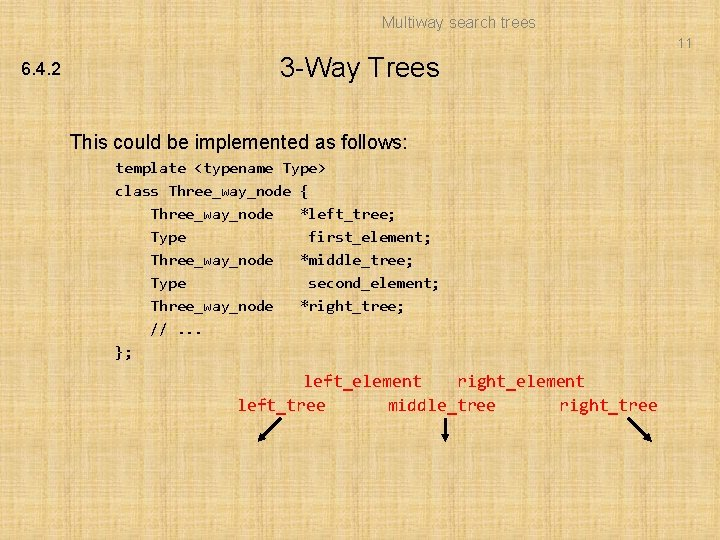Multiway search trees 11 6. 4. 2 3 -Way Trees This could be implemented as follows: template class Three_way_node { Three_way_node *left_tree; Type first_element; Three_way_node *middle_tree; Type second_element; Three_way_node *right_tree; //. . . }; right_element left_element middle_tree left_tree right_tree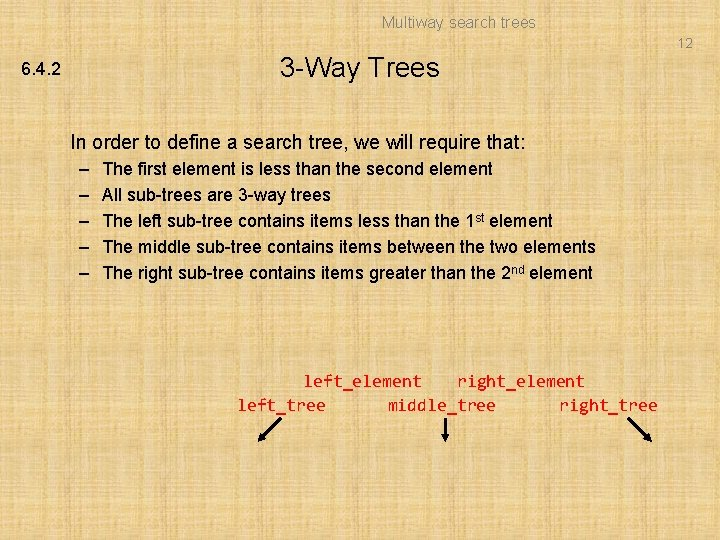Multiway search trees 12 3 -Way Trees 6. 4. 2 In order to define a search tree, we will require that: – – – The first element is less than the second element All sub-trees are 3 -way trees The left sub-tree contains items less than the 1 st element The middle sub-tree contains items between the two elements The right sub-tree contains items greater than the 2 nd element right_element left_element middle_tree left_tree right_treeMultiway search trees 13 3 -Way Trees 6. 4. 2 If a node has only one element, all trees are assumed to be empty – If a second object is inserted, it will be inserted into this node template class Three_way_node { Three_way_node *left_tree; Type first_element; Three_way_node *middle_tree; Type second_element; Three_way_node *right_tree; int num_elements; //. . . # 1 or 2 }; template bool Three_way_node: : full() const { return num_elements == 2; }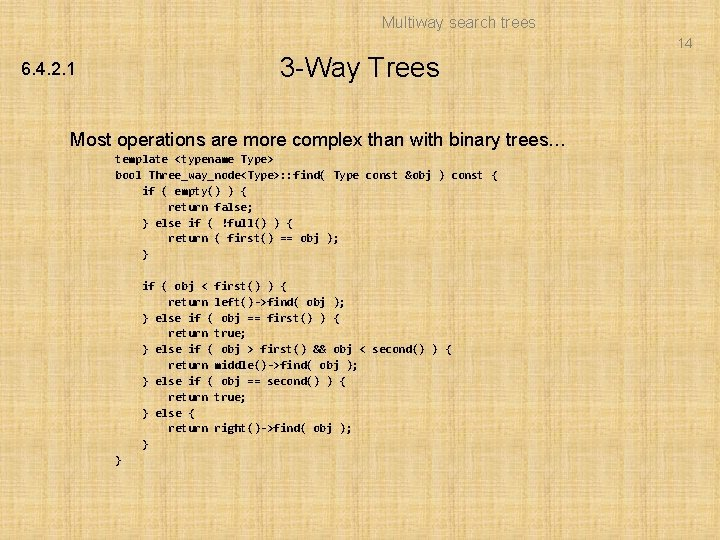Multiway search trees 14 3 -Way Trees 6. 4. 2. 1 Most operations are more complex than with binary trees… template bool Three_way_node: : find( Type const &obj ) const { if ( empty() ) { return false; } else if ( !full() ) { return ( first() == obj ); } if ( obj < first() ) { return left()->find( obj ); } else if ( obj == first() ) { return true; } else if ( obj > first() && obj < second() ) { return middle()->find( obj ); } else if ( obj == second() ) { return true; } else { return right()->find( obj ); } }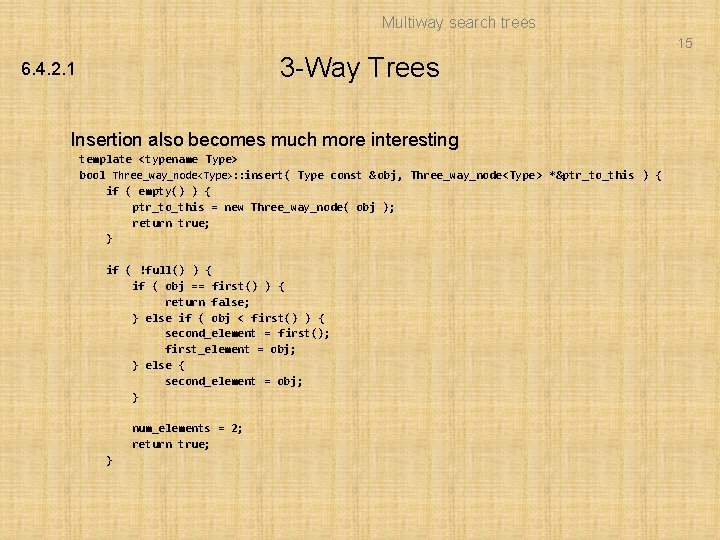Multiway search trees 15 3 -Way Trees 6. 4. 2. 1 Insertion also becomes much more interesting template bool Three_way_node: : insert( Type const &obj, Three_way_node *&ptr_to_this ) { if ( empty() ) { ptr_to_this = new Three_way_node( obj ); return true; } if ( !full() ) { if ( obj == first() ) { return false; } else if ( obj < first() ) { second_element = first(); first_element = obj; } else { second_element = obj; } num_elements = 2; return true; }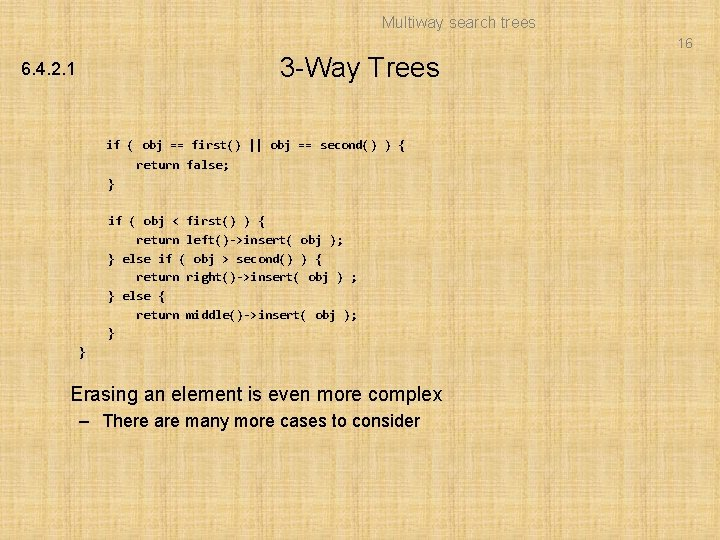Multiway search trees 16 3 -Way Trees 6. 4. 2. 1 if ( obj == first() || obj == second() ) { return false; } if ( obj < first() ) { return left()->insert( obj ); } else if ( obj > second() ) { return right()->insert( obj ) ; } else { return middle()->insert( obj ); } } Erasing an element is even more complex – There are many more cases to considerMultiway search trees 17 6. 4. 2. 2 Insertion into 3 -Way Trees Consider inserting values into an empty 3 -way tree: – Starting with 68, it would be inserted into the rootMultiway search trees 18 6. 4. 2. 2 Insertion into 3 -Way Trees If 27 was inserted next, it would be fit into the root node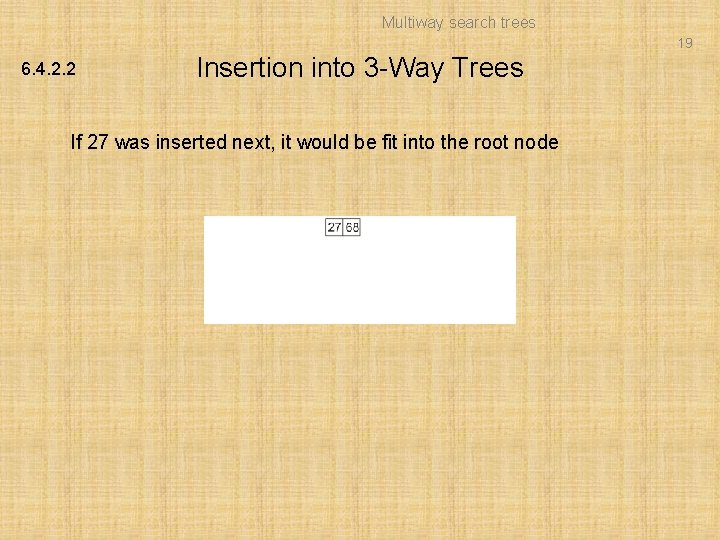Multiway search trees 19 6. 4. 2. 2 Insertion into 3 -Way Trees If 27 was inserted next, it would be fit into the root nodeMultiway search trees 20 6. 4. 2. 2 Insertion into 3 -Way Trees Any new insertion would create an appropriate sub-tree – Inserting 91, we note that 91 > 68, so a right sub-tree is constructed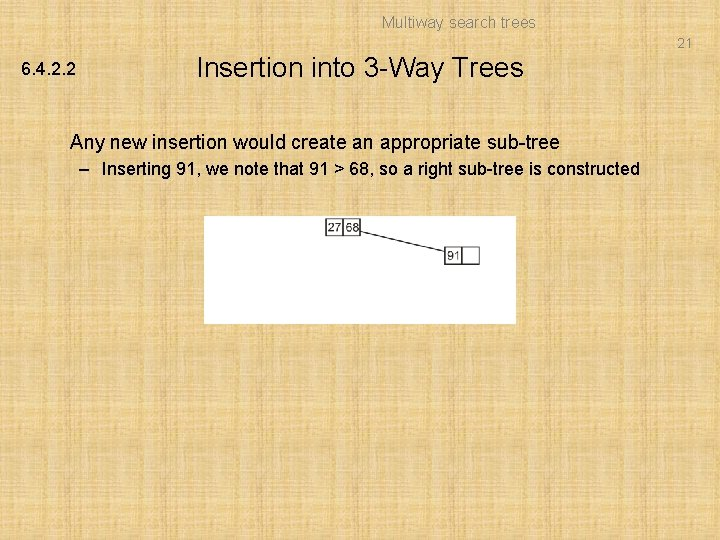Multiway search trees 21 6. 4. 2. 2 Insertion into 3 -Way Trees Any new insertion would create an appropriate sub-tree – Inserting 91, we note that 91 > 68, so a right sub-tree is constructed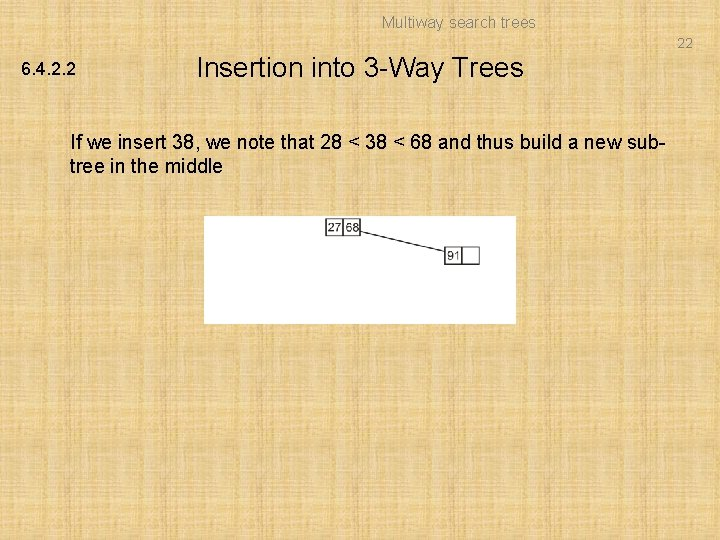Multiway search trees 22 6. 4. 2. 2 Insertion into 3 -Way Trees If we insert 38, we note that 28 < 38 < 68 and thus build a new subtree in the middle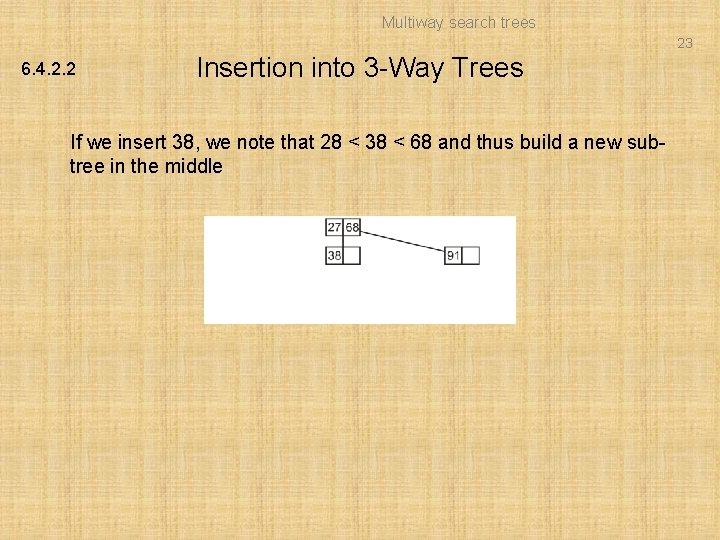Multiway search trees 23 6. 4. 2. 2 Insertion into 3 -Way Trees If we insert 38, we note that 28 < 38 < 68 and thus build a new subtree in the middle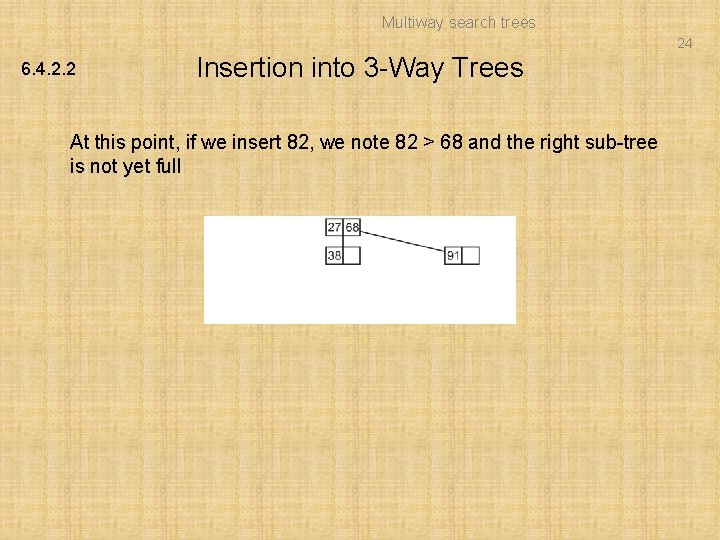Multiway search trees 24 6. 4. 2. 2 Insertion into 3 -Way Trees At this point, if we insert 82, we note 82 > 68 and the right sub-tree is not yet full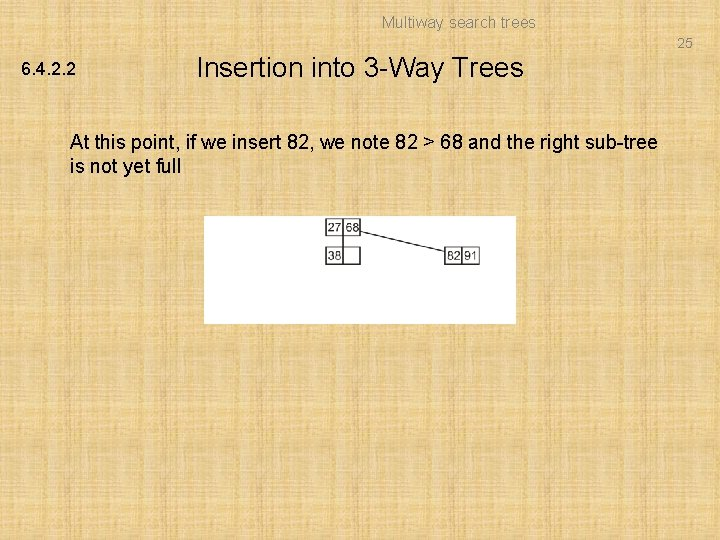Multiway search trees 25 6. 4. 2. 2 Insertion into 3 -Way Trees At this point, if we insert 82, we note 82 > 68 and the right sub-tree is not yet full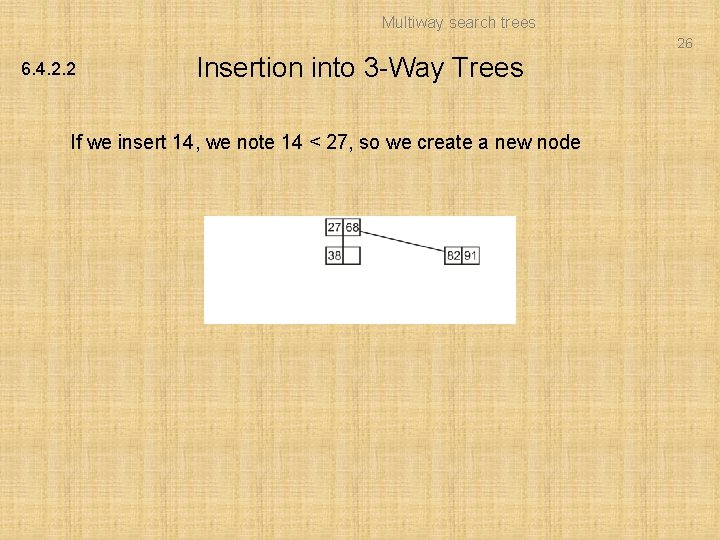Multiway search trees 26 6. 4. 2. 2 Insertion into 3 -Way Trees If we insert 14, we note 14 < 27, so we create a new nodeMultiway search trees 27 6. 4. 2. 2 Insertion into 3 -Way Trees If we insert 14, we note 14 < 27, so we create a new nodeMultiway search trees 28 6. 4. 2. 2 Insertion into 3 -Way Trees Next, inserting 62, 27 < 62 < 28 so we insert it into the middle subtree which also is not full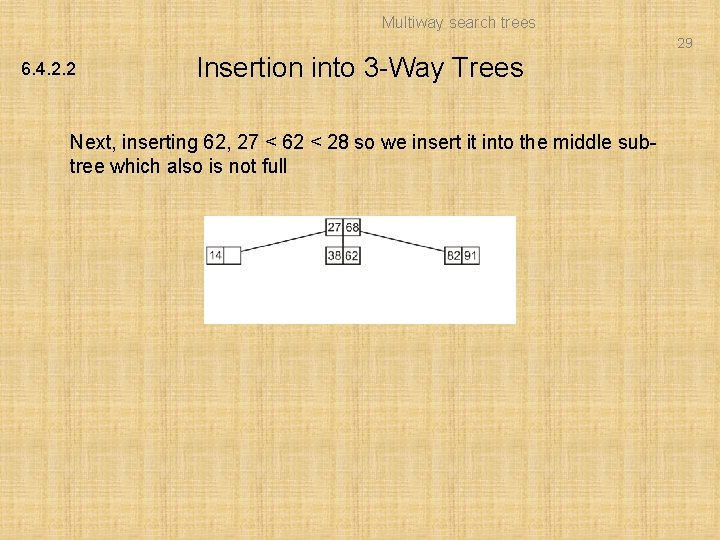Multiway search trees 29 6. 4. 2. 2 Insertion into 3 -Way Trees Next, inserting 62, 27 < 62 < 28 so we insert it into the middle subtree which also is not fullMultiway search trees 30 6. 4. 2. 2 Insertion into 3 -Way Trees If we insert 45, – First, 27 < 45 < 68 and then 38 < 45 < 62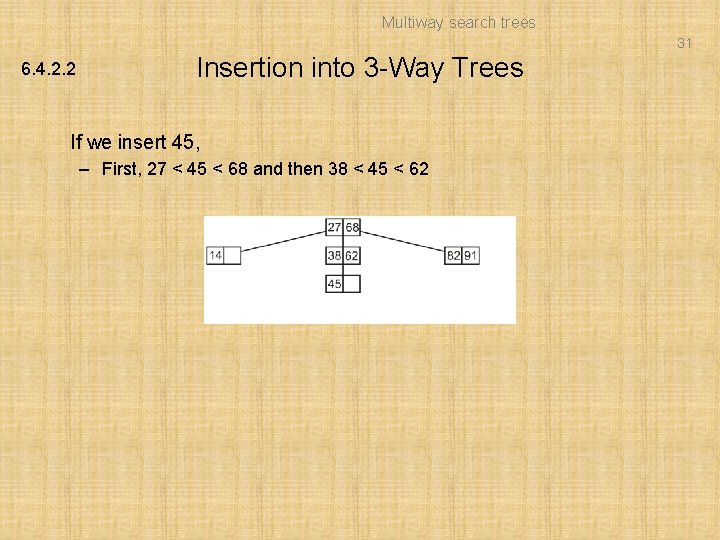Multiway search trees 31 6. 4. 2. 2 Insertion into 3 -Way Trees If we insert 45, – First, 27 < 45 < 68 and then 38 < 45 < 62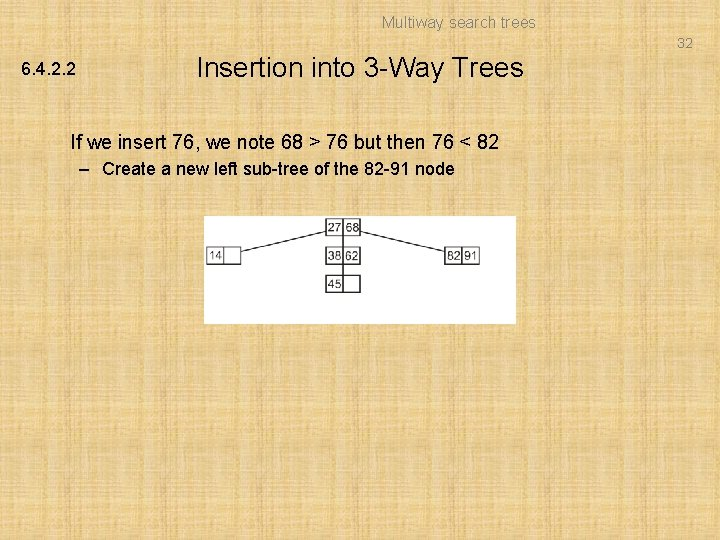Multiway search trees 32 6. 4. 2. 2 Insertion into 3 -Way Trees If we insert 76, we note 68 > 76 but then 76 < 82 – Create a new left sub-tree of the 82 -91 node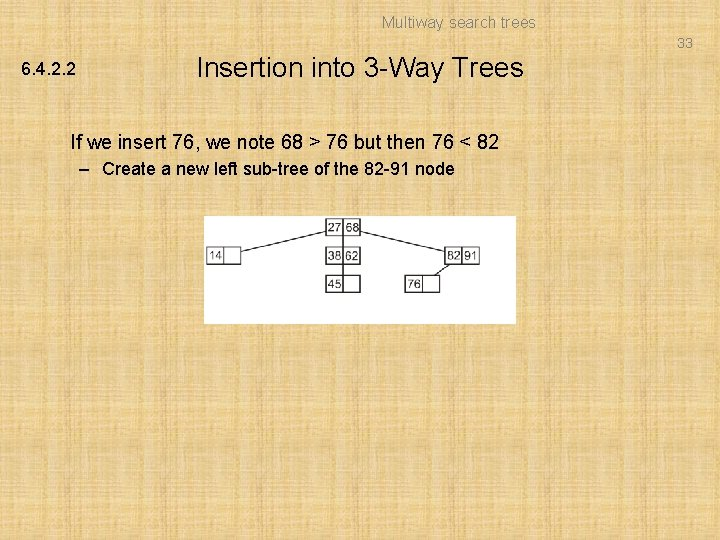Multiway search trees 33 6. 4. 2. 2 Insertion into 3 -Way Trees If we insert 76, we note 68 > 76 but then 76 < 82 – Create a new left sub-tree of the 82 -91 nodeMultiway search trees 34 6. 4. 2. 2 Insertion into 3 -Way Trees If we insert 4, 4 < 27 and the left sub-tree contains only a single element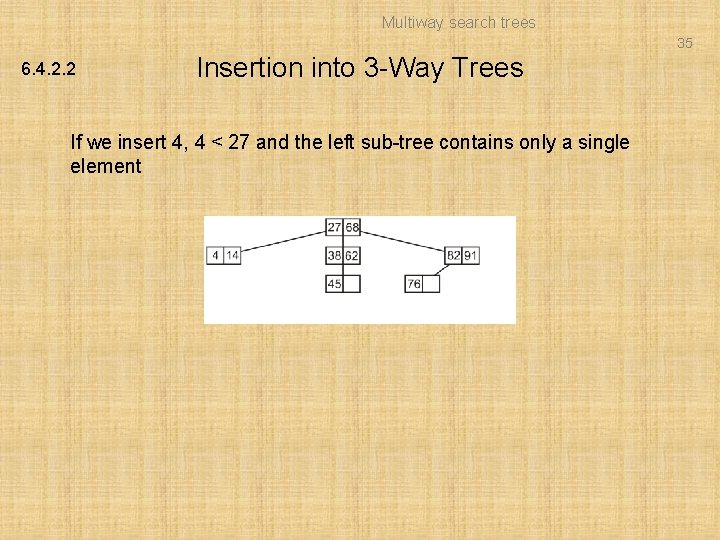Multiway search trees 35 6. 4. 2. 2 Insertion into 3 -Way Trees If we insert 4, 4 < 27 and the left sub-tree contains only a single element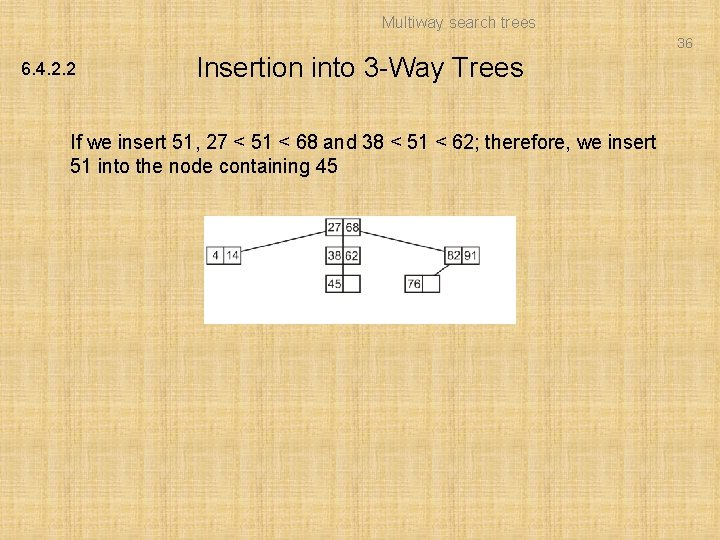Multiway search trees 36 6. 4. 2. 2 Insertion into 3 -Way Trees If we insert 51, 27 < 51 < 68 and 38 < 51 < 62; therefore, we insert 51 into the node containing 45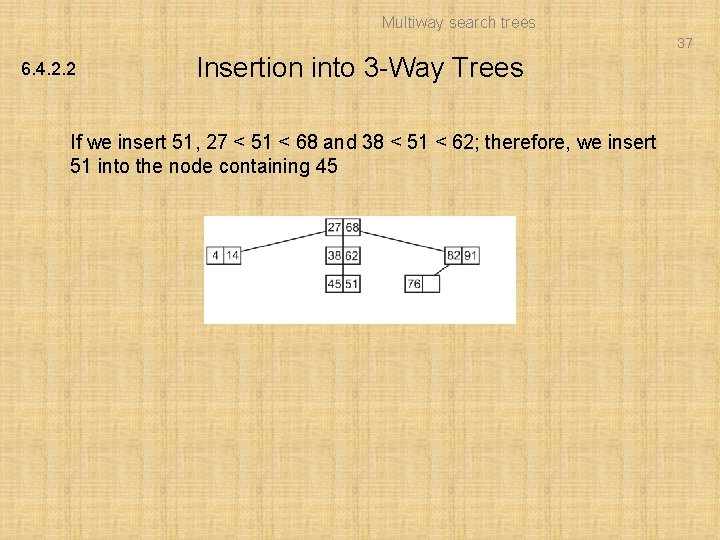Multiway search trees 37 6. 4. 2. 2 Insertion into 3 -Way Trees If we insert 51, 27 < 51 < 68 and 38 < 51 < 62; therefore, we insert 51 into the node containing 45Multiway search trees 38 6. 4. 2. 2 Insertion into 3 -Way Trees If we insert 8, 8 < 27 and then 4 < 8 < 14 – Construct a new middle sub-tree of the 4 -14 node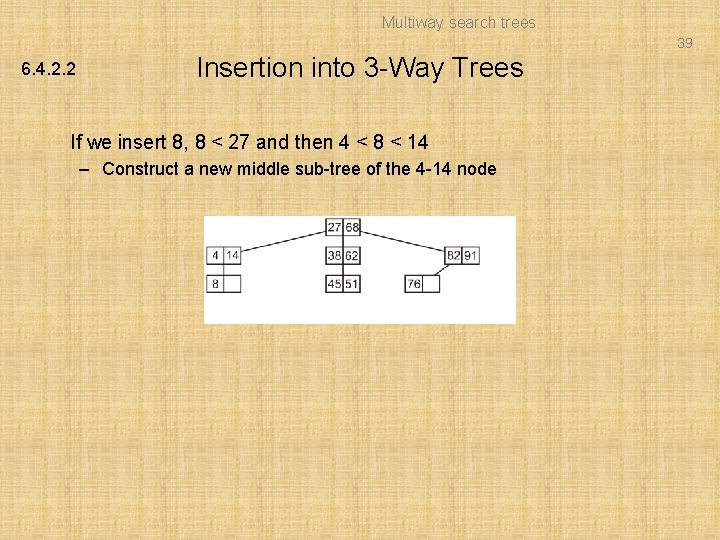Multiway search trees 39 6. 4. 2. 2 Insertion into 3 -Way Trees If we insert 8, 8 < 27 and then 4 < 8 < 14 – Construct a new middle sub-tree of the 4 -14 nodeMultiway search trees 40 6. 4. 2. 2 Insertion into 3 -Way Trees If we insert 98, 98 > 68 and 98 > 91 – Construct a new right sub-tree of the 81 -91 node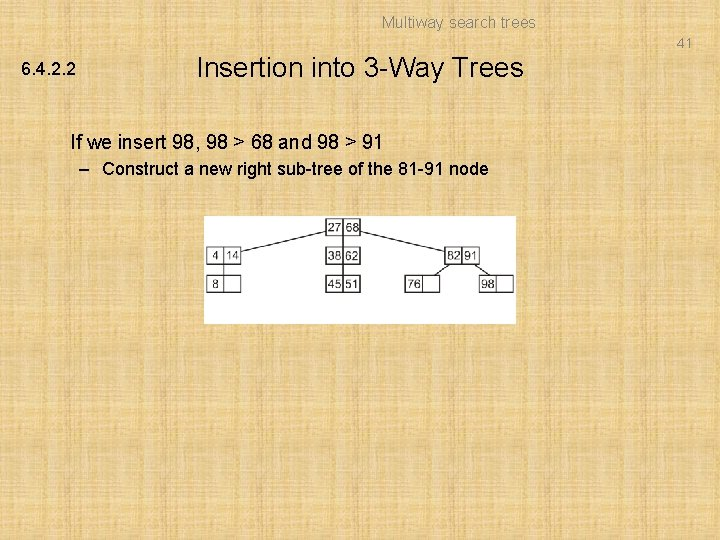Multiway search trees 41 6. 4. 2. 2 Insertion into 3 -Way Trees If we insert 98, 98 > 68 and 98 > 91 – Construct a new right sub-tree of the 81 -91 node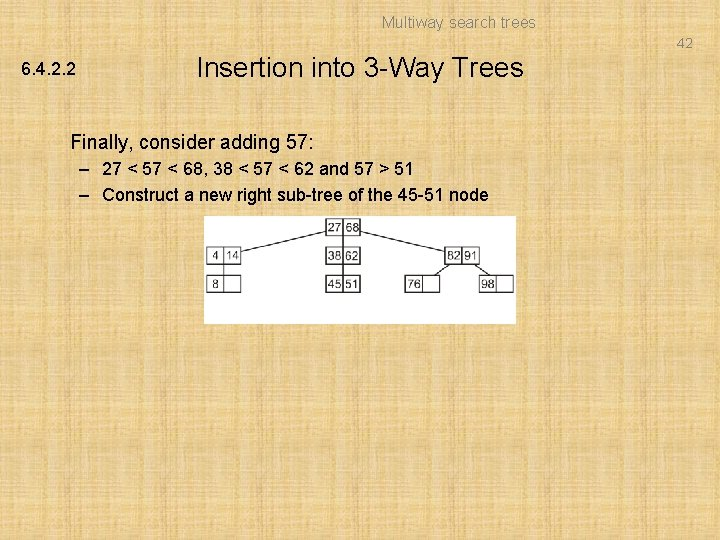Multiway search trees 42 6. 4. 2. 2 Insertion into 3 -Way Trees Finally, consider adding 57: – 27 < 57 < 68, 38 < 57 < 62 and 57 > 51 – Construct a new right sub-tree of the 45 -51 node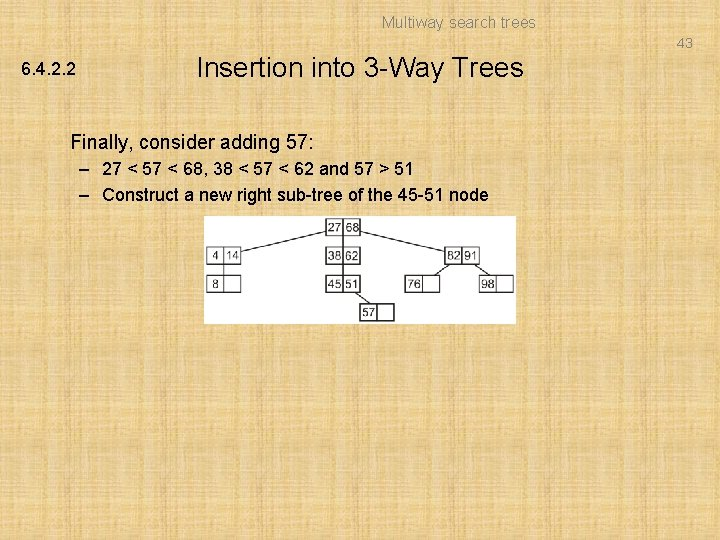Multiway search trees 43 6. 4. 2. 2 Insertion into 3 -Way Trees Finally, consider adding 57: – 27 < 57 < 68, 38 < 57 < 62 and 57 > 51 – Construct a new right sub-tree of the 45 -51 node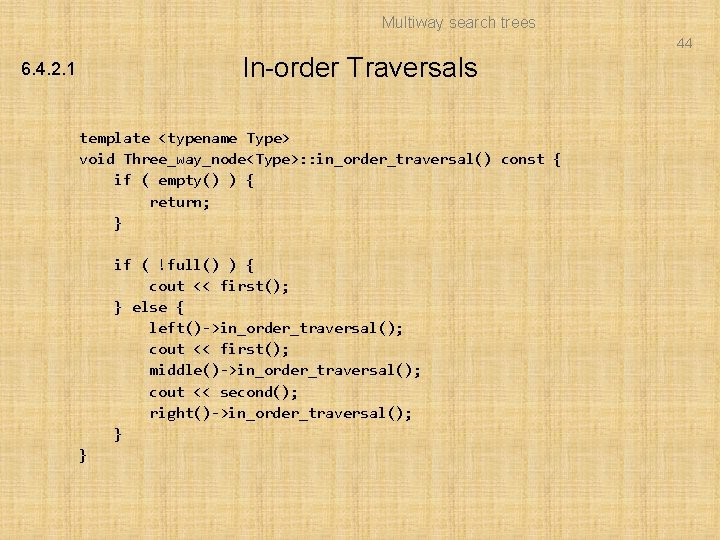Multiway search trees 44 In-order Traversals 6. 4. 2. 1 template void Three_way_node: : in_order_traversal() const { if ( empty() ) { return; } if ( !full() ) { cout << first(); } else { left()->in_order_traversal(); cout << first(); middle()->in_order_traversal(); cout << second(); right()->in_order_traversal(); } }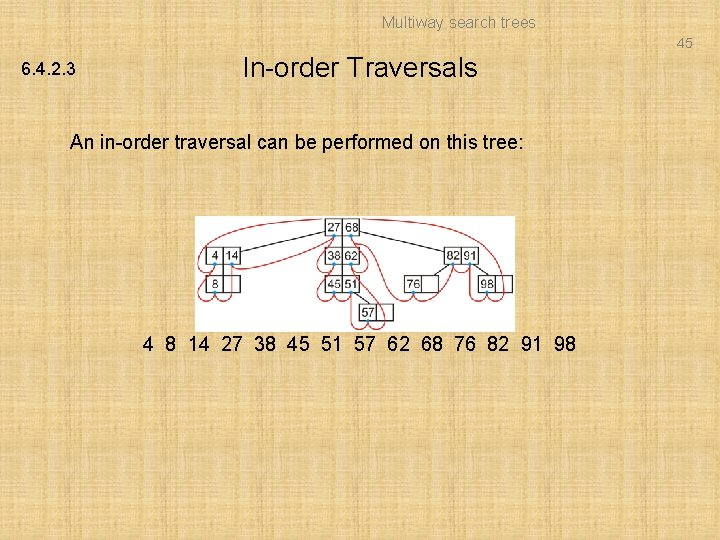Multiway search trees 45 6. 4. 2. 3 In-order Traversals An in-order traversal can be performed on this tree: 4 8 14 27 38 45 51 57 62 68 76 82 91 98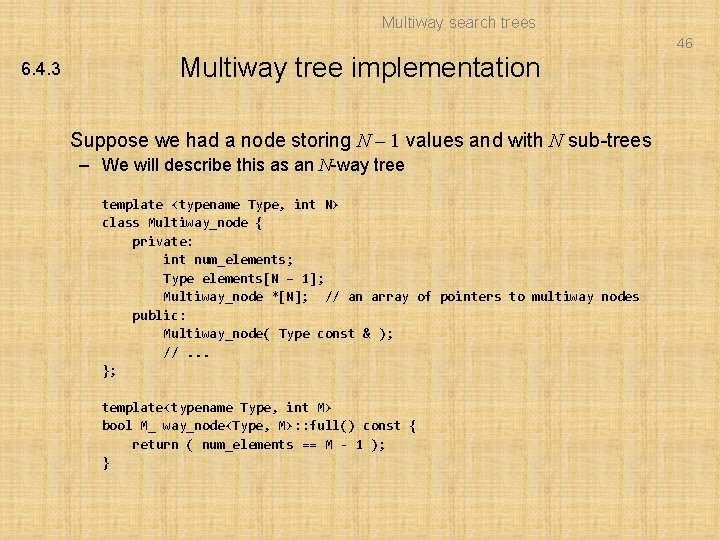Multiway search trees 46 6. 4. 3 Multiway tree implementation Suppose we had a node storing N – 1 values and with N sub-trees – We will describe this as an N-way tree template class Multiway_node { private: int num_elements; Type elements[N – 1]; Multiway_node *[N]; // an array of pointers to multiway nodes public: Multiway_node( Type const & ); //. . . }; template bool M_ way_node: : full() const { return ( num_elements == M - 1 ); }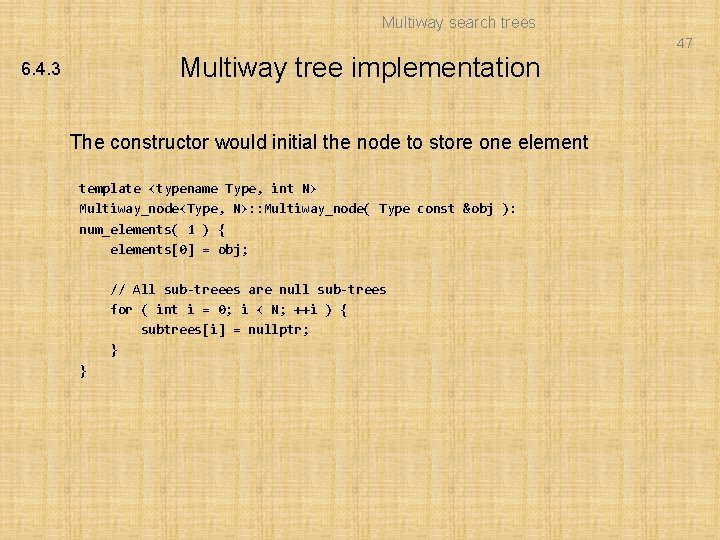Multiway search trees 47 Multiway tree implementation 6. 4. 3 The constructor would initial the node to store one element template Multiway_node: : Multiway_node( Type const &obj ): num_elements( 1 ) { elements = obj; // All sub-treees are null sub-trees for ( int i = 0; i < N; ++i ) { subtrees[i] = nullptr; } }Multiway search trees 48 Multiway tree implementation 6. 4. 3 An in-order traversal would be similar: template void Multiway_node: : in_order_traversal() const { if ( empty() ) { return; } else if ( !full() ) { for ( int i = 0; i < num_elements; ++i ) { cout << elements[i]; } } else { for ( int i = 0; i < N - 1; ++i ) { subtrees[i]->in_order_traversal(); cout << elements[i]; } subtrees[N - 1]->in_order_traversal(); } }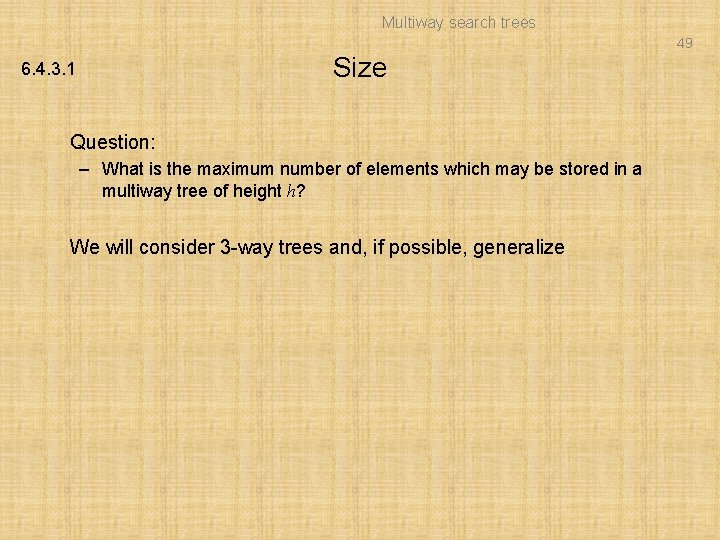Multiway search trees 49 Size 6. 4. 3. 1 Question: – What is the maximum number of elements which may be stored in a multiway tree of height h? We will consider 3 -way trees and, if possible, generalizeMultiway search trees 50 Size 6. 4. 3. 1 Examining these perfect 3 -way trees we get the table: h Size Formula 0 2 31 – 1 1 8 32 – 1 2 26 33 – 1 3 80 34 – 1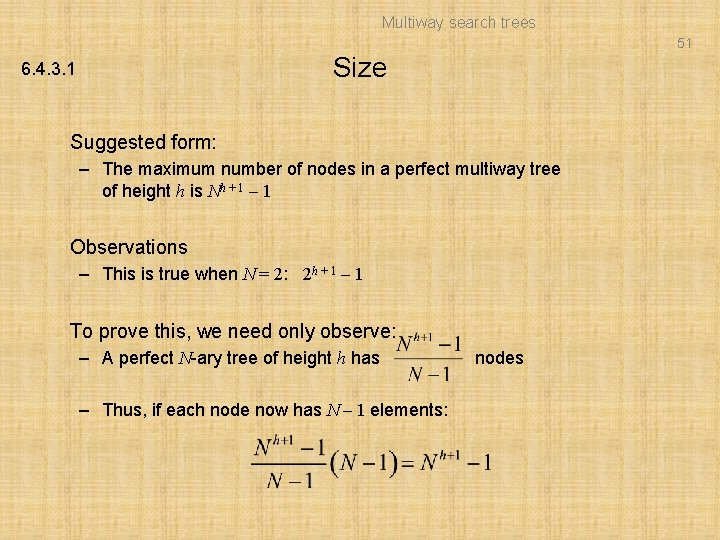Multiway search trees 51 Size 6. 4. 3. 1 Suggested form: – The maximum number of nodes in a perfect multiway tree of height h is Nh + 1 – 1 Observations – This is true when N = 2: 2 h + 1 – 1 To prove this, we need only observe: – A perfect N-ary tree of height h has – Thus, if each node now has N – 1 elements: nodes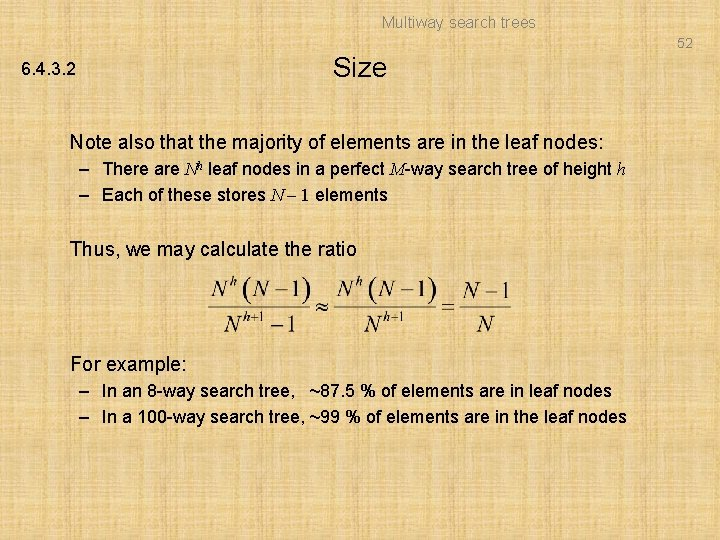Multiway search trees 52 Size 6. 4. 3. 2 Note also that the majority of elements are in the leaf nodes: – There are Nh leaf nodes in a perfect M-way search tree of height h – Each of these stores N – 1 elements Thus, we may calculate the ratio For example: – In an 8 -way search tree, ~87. 5 % of elements are in leaf nodes – In a 100 -way search tree, ~99 % of elements are in the leaf nodes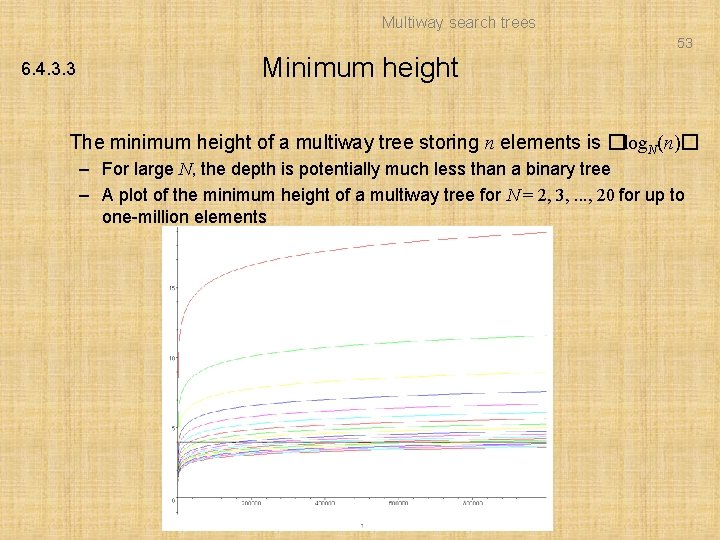Multiway search trees 53 6. 4. 3. 3 Minimum height The minimum height of a multiway tree storing n elements is �log. N(n)� – For large N, the depth is potentially much less than a binary tree – A plot of the minimum height of a multiway tree for N = 2, 3, . . . , 20 for up to one-million elements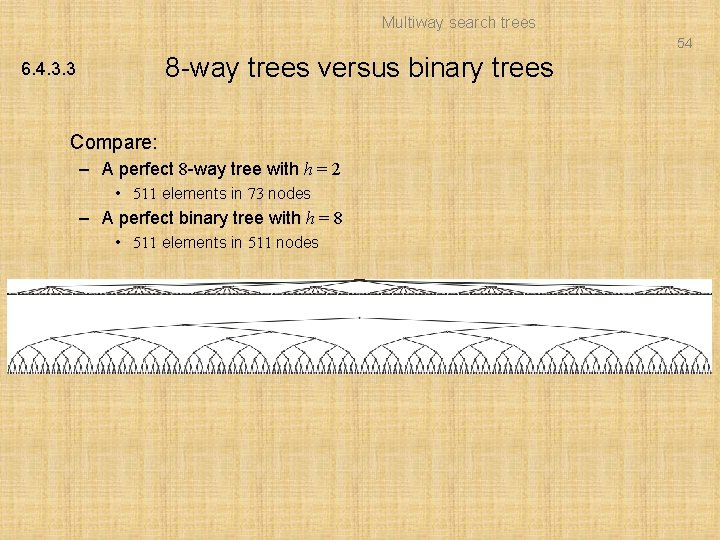Multiway search trees 54 8 -way trees versus binary trees 6. 4. 3. 3 Compare: – A perfect 8 -way tree with h = 2 • 511 elements in 73 nodes – A perfect binary tree with h = 8 • 511 elements in 511 nodes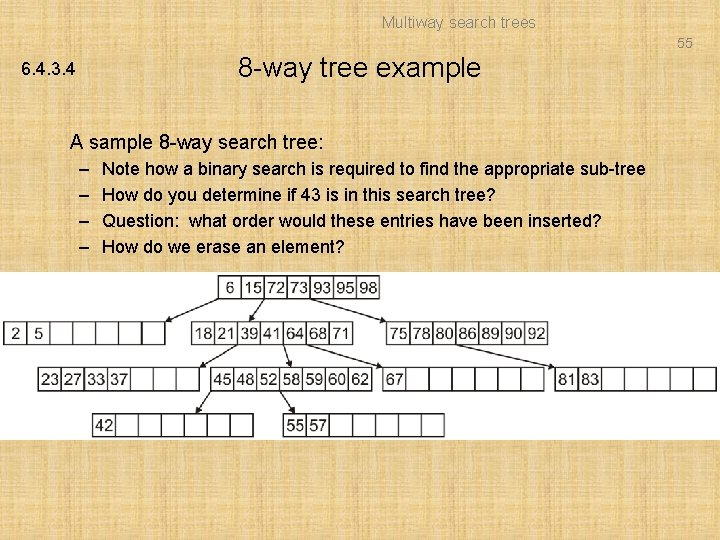Multiway search trees 55 8 -way tree example 6. 4. 3. 4 A sample 8 -way search tree: – – Note how a binary search is required to find the appropriate sub-tree How do you determine if 43 is in this search tree? Question: what order would these entries have been inserted? How do we erase an element?Multiway search trees 56 Multiway trees 6. 4. 3. 4 Advantage: – Shorter paths from the root Disadvantage: – More complex Under what conditions is the additional complexity worth the effort? – When the cost from jumping nodes is exceptionally dominant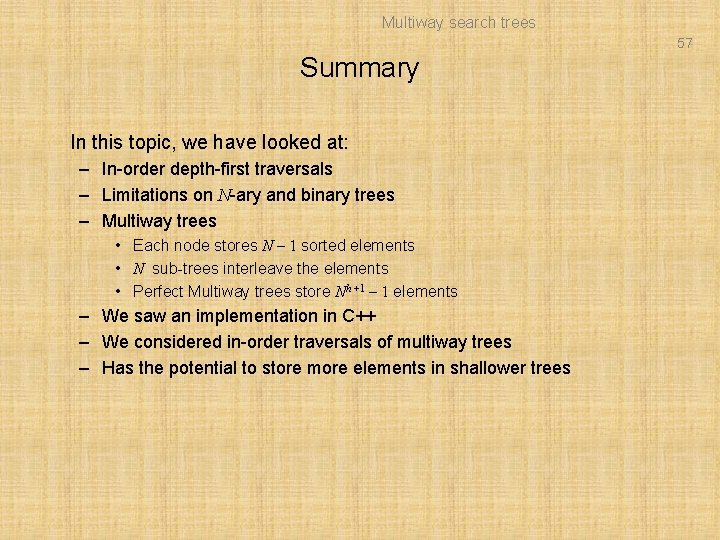Multiway search trees 57 Summary In this topic, we have looked at: – In-order depth-first traversals – Limitations on N-ary and binary trees – Multiway trees • Each node stores N – 1 sorted elements • N sub-trees interleave the elements • Perfect Multiway trees store Nh +1 – 1 elements – We saw an implementation in C++ – We considered in-order traversals of multiway trees – Has the potential to store more elements in shallower treesMultiway search trees 58 References  Cormen, Leiserson, and Rivest, Introduction to Algorithms, MIT Press, 1990, § 7. 1 -3, p. 152.  Weiss, Data Structures and Algorithm Analysis in C++, 3 rd Ed. , Addison Wesley, § 6. 5 -6, p. 215 -25.Multiway search trees 59 Usage Notes • These slides are made publicly available on the web for anyone to use • If you choose to use them, or a part thereof, for a course at another institution, I ask only three things: – that you inform me that you are using the slides, – that you acknowledge my work, and – that you alert me of any mistakes which I made or changes which you make, and allow me the option of incorporating such changes (with an acknowledgment) in my set of slides Sincerely, Douglas Wilhelm Harder, MMath [email protected] uwaterloo. ca